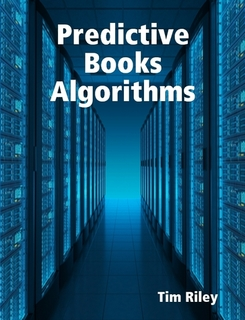Predictive Books Algorithms"Predictive Books Algorithms" is an accounting math book. Also available is the corresponding "Predictive Books Examples." Paperback versions of these books are available at a nominal cost.

"Predictive Books Algorithms" helps accountants model a firm's economic events. Economic events are observed and then processed through a highly defined algorithm. The basic algorithm begins with the identity:

Assets = Liabilities + Owners' Equity

Each Asset, Liability, and Owners' Equity expands to many accounting subclassifications. Each subclassification further expands to many accounts. After an economic event is observed, the appropriate accounting algorithm is mathematically applied. The output from the algorithm is a journal entry.